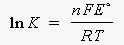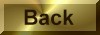Practice Problem 12

Use the relationship betweenGo and Eo for an electrochemical reaction to derive the relationship between the standard-state cell potential and the equilibrium constant for the reaction.

We can start with the equation that describes the relationship between the standard-state free energy of reaction and the standard-state cell potential for an electrochemical cell:Go = -nFEo

We can then write the relationship between the standard-state free energy fo reaction and the equilibrium constant for the reaction:Go = - RT ln K

Substituting one of these equations into the other gives:

-nFE= - RT ln K

We now multiply both sides of this equation by -1:

nFE= RT ln K

Solving for the ln K term gives the following equation: